• 对数的线性回归方程
万次阅读
2017-08-24 10:38:11

墙裂推荐阅读：ｙ的衍生物

关键词：最小二乘法；正则化；对数线性回归； y的衍生物

## 3.1 基本形式

假设样本x有d个属性，线性模型（linear model）试图学得一个通过属性的线性组合来进行预测的函数，即 f(x)=w1x1+w2x2++wdxd+b f ( x ) = w 1 x 1 + w 2 x 2 + ⋅ ⋯ + w d x d + b $f(x)=w_{1}x_{1} + w_{2}x_{2}+\cdot \cdots +w_{d}x_{d}+b$ ，向量形式 f(x)=wTx+b f ( x ) = w T x + b $f(x)=w^{T}x+b$

## 3.2 线性回归

关键词：无序属性连续化。
对离散属性，若属性值之间存在“序”（order）关系，可通过连续化将其转化为连续值，例如二值属性身高的取值，“高”“矮”可和转化为｛1.0 ， 0｝。 若属性值之间不存在序的关系，例如属性“瓜类”的取值为西瓜，南瓜，冬瓜，则可转化为（0，0，1），（0，1，0），（1，0，0）。

关键词：最小二乘法（least square method）。
基于均方误差最小化来进行模型求解的方法称为“最小二乘法”。在线性回归中，最小二乘法就是试图找到一条直线，使所有样本到直线上的欧氏距离之和最小。

关键词： 正则化（regularization）项。
假设解一个线性方程组，当方程数大于自由变量数时，是没有解的。反过来，当方程数小于自由变量数的时候，解就有很多个了。往往，我们会碰到这种情况，参数多，“方程”少的情况，那么有很多个w（权值向量）都能使均方误差最小，那么该选哪一个呢？ 这就涉及到 归纳偏好问题了，常见的做法是引入正则化项。

关键词：对数线性回归（log-linear regression）；y的衍生物
把线性回归模型简写为： f(x)=wTx+b f ( x ) = w T x + b $f(x)=w^{T}x+b$ ，当我们希望线性模型的预测值逼近真实标记y，这样就是线性模型。那可否令模型的预测值毕竟y的衍生物呢？ 作者的这一描述实在太妙了！y的衍生物，通俗易懂！ 假设y的衍生物是 y的对数即lny，那么就可以得到对数线性回归模型： lny=wTx+b l n y = w T x + b $lny=w^{T}x+b$ ， 也就是让模型 去逼近 lny，而不是y。也可以对 lny=wTx+b l n y = w T x + b $lny=w^{T}x+b$ 做一下变换就变成了 y=ewTx+b y = e w T x + b $y=e^{w^{T}x+b}$，也可以理解为让 ewTx+b e w T x + b $e^{w^{T}x+b}$ 去逼近y。形式上还是线性回归的，但实质上已是在求取输入空间到输出空间的非线性函数映射。如图：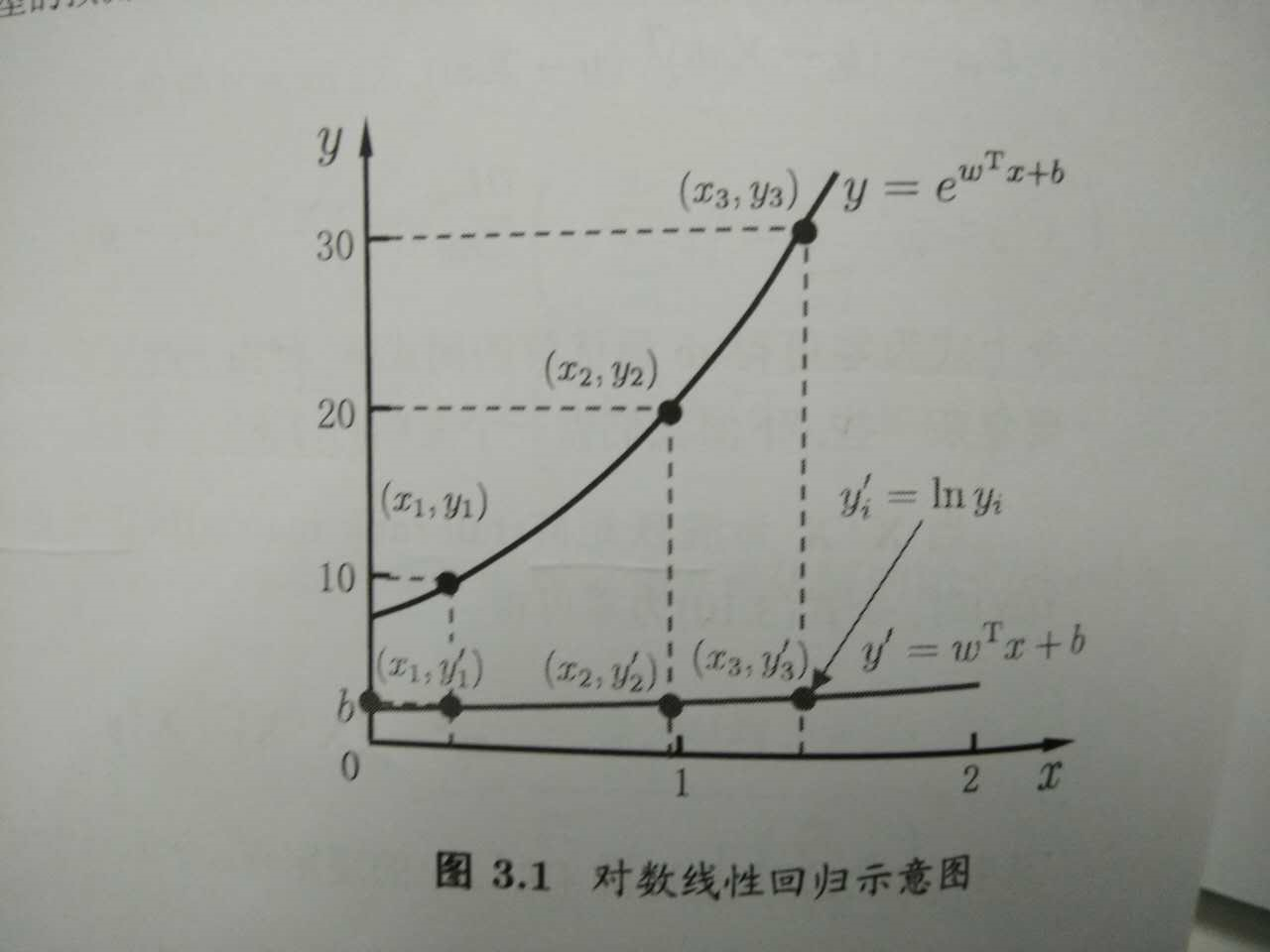来思考一个问题
想从线性模型出发，去扩展线性模型，就是让线性模型 f(x)=wTx+b f ( x ) = w T x + b $f(x)=w^{T}x+b$去拟合y的衍生物，那么我们常说的逻辑回归（对数几率回归）是怎么从线性模型演变而来的呢？是让 wTx+b w T x + b $w^{T}x+b$去拟合哪一种“ｙ的衍生物” 什么呢？这个可以思考思考后，请看下篇：逻辑回归机器学习 线性回归
更多相关内容
• 使用方法： 首先输入实验数据的对数 （一个x和一个y算一对） 然后输入x值和y值 全部输入结束时会被询问是否修改实验数据。如果修改，输入y，否则输入n 然后输入B类不确定度，随后显示最终结果exe格式
• 如果是分类任务，如何使用线性回归模型呢？答案在广义线性模型的公式中，只需要找到一个单调可微函数将分类任务的真实标记y 与线性回归模型的预测值联系起来。 考虑二分类任务，输出 $y \in${0,1} , 线性回归的预测...

# 对数几率回归

又常常称为逻辑回归逻辑斯谛回归

如果是分类任务，如何使用线性回归模型呢？答案在广义线性模型的公式中，只需要找到一个单调可微函数将分类任务的真实标记y 与线性回归模型的预测值联系起来

考虑二分类任务，输出 y ∈ { 0 , 1 } y \in \{0,1\} , 线性回归的预测值 z = w T x + b z=w^Tx + b 是实值，需要对 z 进行转化，最理想的转换函数是单位阶跃函数，即预测值大于0时，判断正，小于0，判断负，等于0，则随机。但该函数是分段函数，是不连续函数，不符合广义线性模型中“联系函数”的要求，对数几率函数形似单位阶跃函数，但它是连续单调可微的。对数几率函数是一种“Sigmoid函数”，它预测值 z 转化为一个接近0或1的 y 值。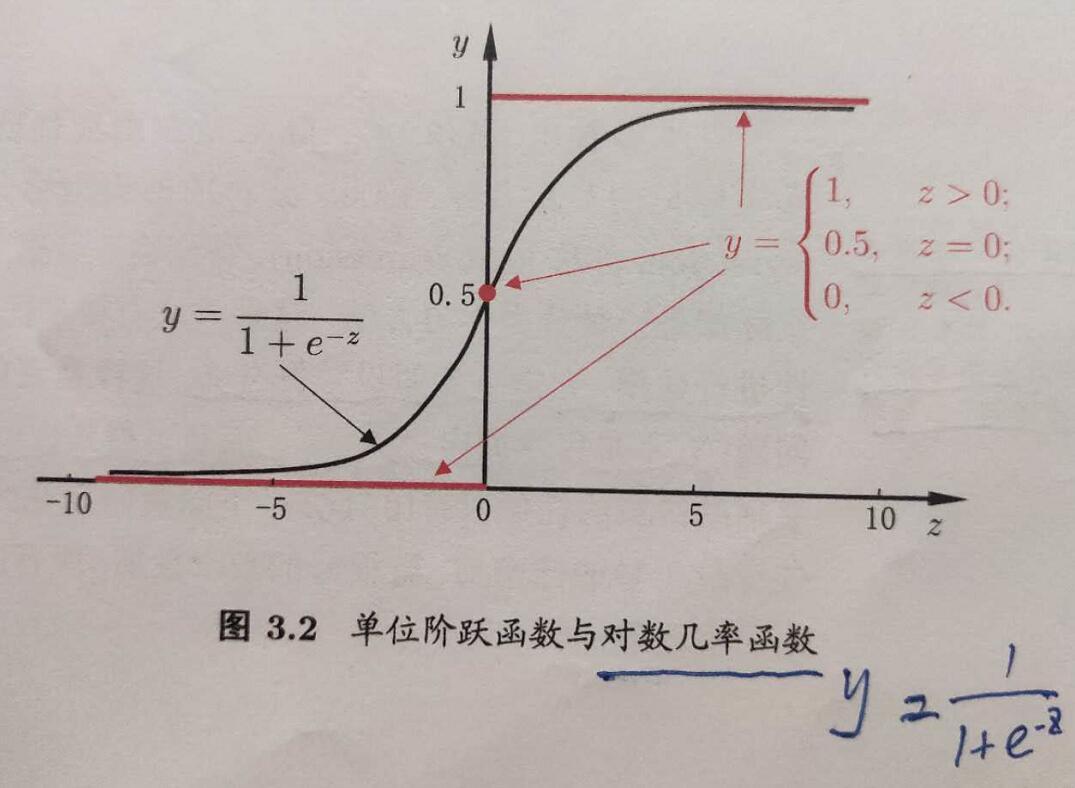y = 1 1 + e − z y = \frac{1}{1 + e^{-z}}

代入广义线性模型公式得到

y = 1 1 + e − ( w T x + b ) y = \frac{1}{1 + e^{-(w^Tx + b)}}

上述公式就是对数几率回归的公式。

这种分类方法有很多优点：它直接对分类可能性进行建模，无需事先假设数据分布；另外，不仅预测出“类别”，还得到了近似概率预测。它的目标函数是任意阶可导的凸函数，有很多数值优化算法，如牛顿法，拟牛顿法等都可用于求取最优解。

根据在线性回归中的讨论，对数几率回归公式可以写成如下形式(推导过程就不写了)：

ln ⁡ y 1 − y = w T x + b \ln\frac{y}{1-y} = w^Tx + b

y 视作类后验概率估计 p ( y = 1 ∣ x ) p(y=1|x) , 上式写作

ln ⁡ p ( y = 1 ∣ x ) p ( y = 0 ∣ x ) = w T x + b \ln\frac{p(y=1|x)}{p(y=0|x)} = w^Tx + b

再加上两个概率和等于1的条件，显然有

p ( y = 1 ∣ x ) = e w T x + b 1 + e w T x + b p(y=1|x) = \frac{e^{w^Tx + b}}{1 + e^{w^Tx + b}}

p ( y = 0 ∣ x ) = 1 1 + e w T x + b p(y=0|x) = \frac{1}{1 + e^{w^Tx + b}}

可通过“极大似然法”来估计 w 和 b 。

l ( w , b ) = ∑ i = 1 m l n p ( y i ∣ x i ; w , b ) = ∑ i = 1 m l n p ( y i ∣ x i ; w , b ) l(w, b) = \sum_{i=1}^m lnp(y_i|x_i; w, b)=\sum_{i=1}^m lnp(y_i|x_i; w, b)

上式就是对数几率回归的目标函数，最大化上述式子，可以通过梯度下降法、牛顿法等来求最优解。

展开全文• ## 多元线性回归方程原理及其推导

万次阅读 多人点赞 2018-10-21 14:19:22
1.在统计学中，线性回归方程是利用最小二乘函数对一个或多个自变量之间关系进行建模的一种回归分析。这种函数是一个或多个称为回归系数的模型参数的线性组合。只有一个自变量的情况称为简单回归，大于一个自变量的...

# 多元线性方程原理及推导

## 概念

1.在统计学中，线性回归方程是利用最小二乘函数对一个或多个自变量之间关系进行建模的一种回归分析。这种函数是一个或多个称为回归系数的模型参数的线性组合。只有一个自变量的情况称为简单回归，大于一个自变量的情况叫多元回归。
2.在线性回归中，数据使用线性预测函数来建模，并且未知的函数模型参数也是通过数据来估计。这种模型被叫做线性模型。最常用的线性回归建模是给定的X的条件下y的条件分布的分位数作为X的线性函数表示。像所有形式的回归分析一样，线性回归也是把焦点放在给定X的值的y的条件概率分布，而不是X和y的联合概率分布（多元分析领域）。

### 公式

一元线性回归方程：y=a+bx
a称为截距
b为回归直线的斜率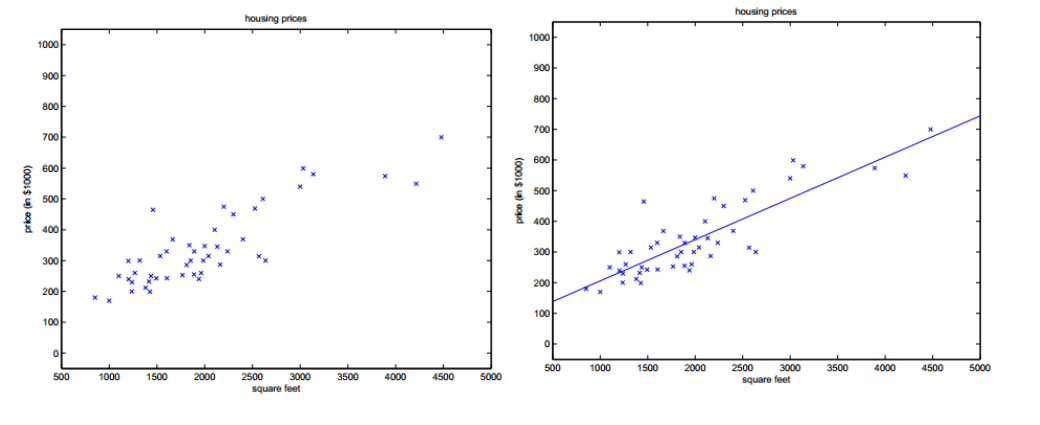多元线性回归方程：y=b0+b1X1+b2X2+…+bnXn
b0为常数项
b1,b2,b3,…bn称为y对应于x1,x2,x3,…xn的偏回归系数

### 推导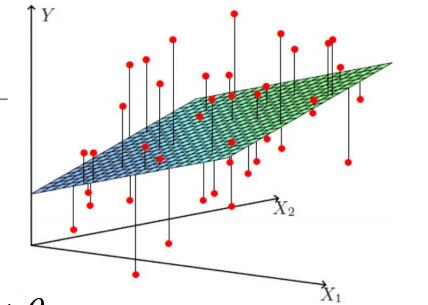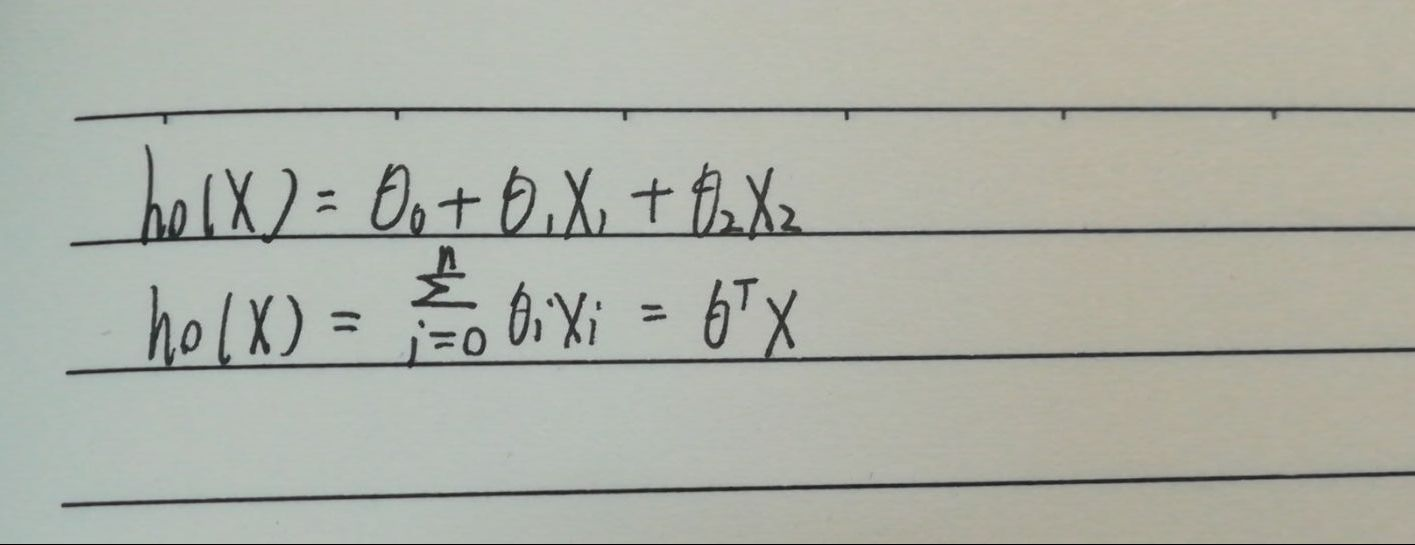使用极大函数解释最小二乘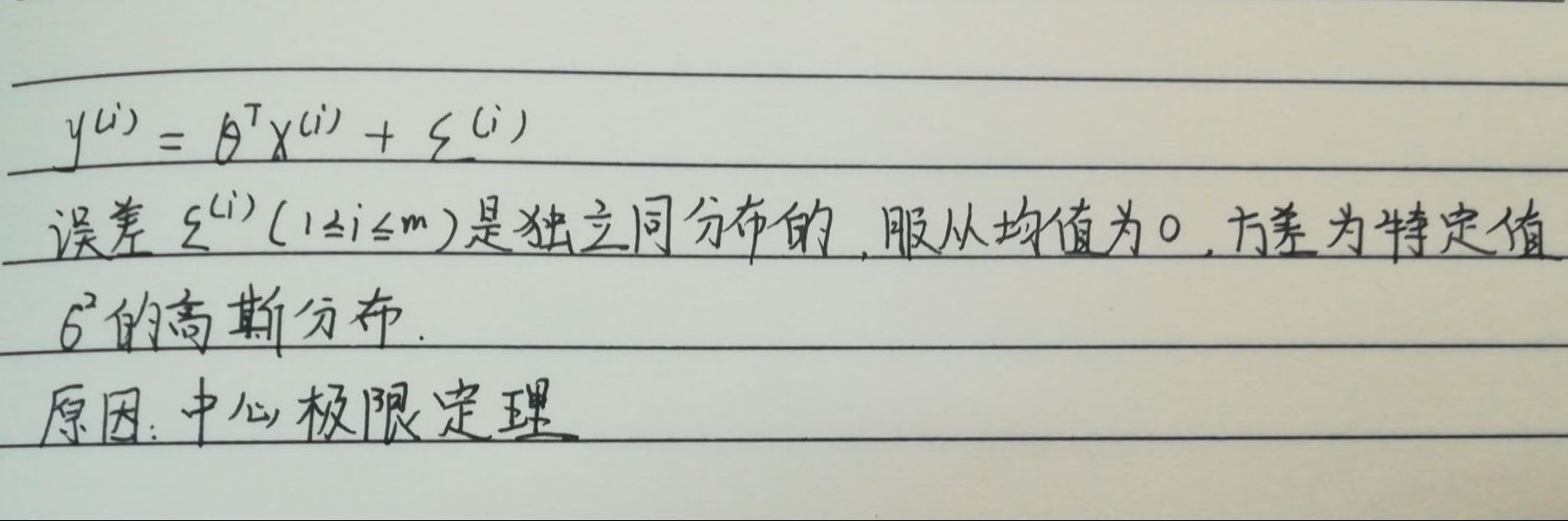似然函数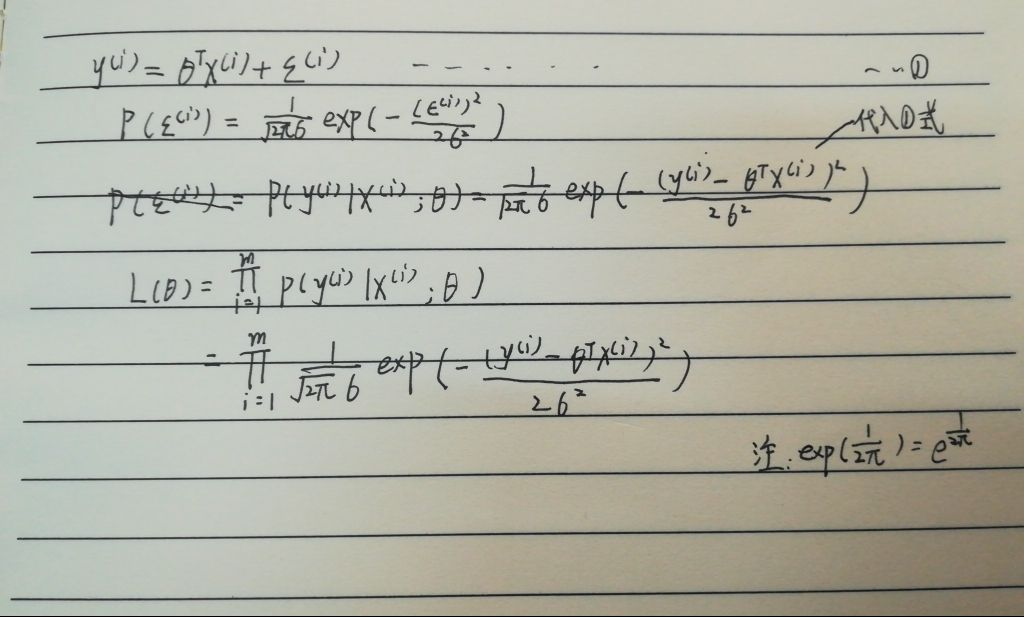高斯的对数似然与最小二乘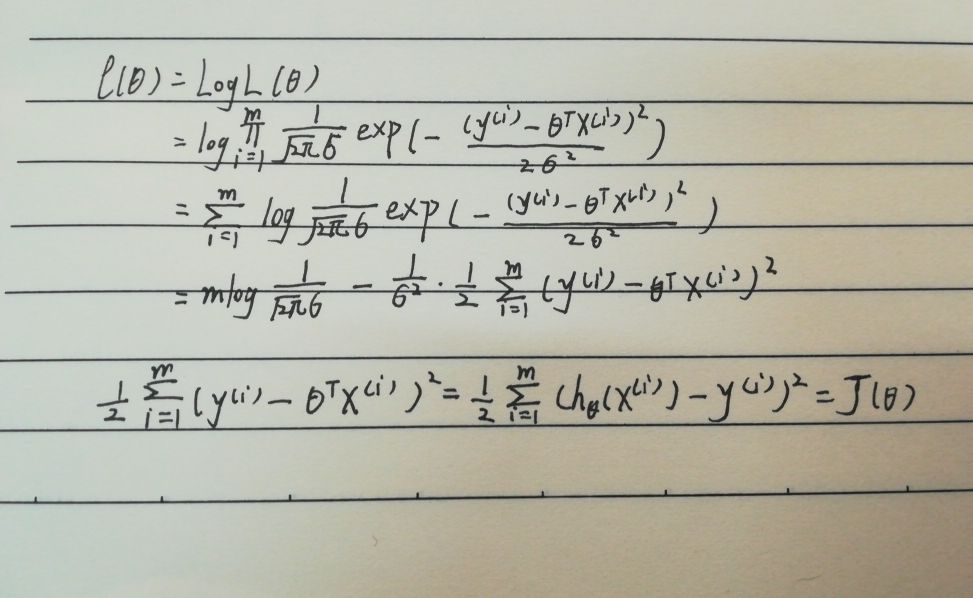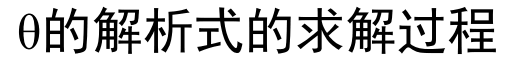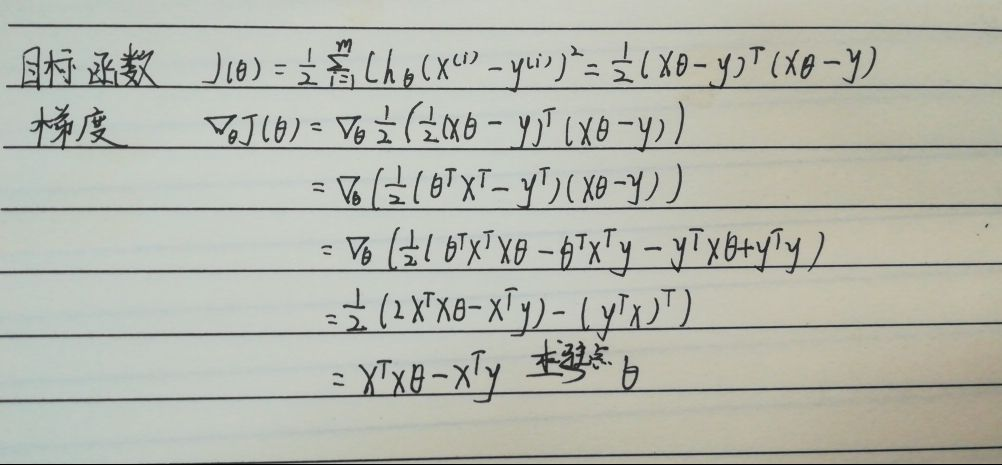参数的解析式#### 总结

    自我认为多元线性方程的推导过程还是挺麻烦的，需要多看几遍。学习推导的过程中，我们要认识一些数学符号，一些方法，比如最小二乘，极大似然，梯度等等。
事实上，一种现象常常是与多个因素相联系的（比如：房子总价与房子面积，房间数，地段这些因素相关），由多个自变量的最优组合共同来预测或估计因变量，比只用一个自变量进行预测或估计更有效，更符合实际。因此多元线性回归比一元线性回归的实用意义更大。

展开全文线性回归 多元线性回归推导
• 首先输入实验数据的对数 （一个x和一个y算一对） 然后输入x值和y值 全部输入结束时会被询问是否修改实验数据。如果修改，输入y，否则输入n 然后输入B类不确定度，随后显示最终结果 如有问题或建议，请和我联系：...
• 对数几率回归（logistic regression），有时候会译为逻辑回归(音译)，其实是我们把回归模型应用到分类问题时，线性回归的一种变形，主要是针对二分类提出的。既然是线性回归的一种变形，那么在理解对数几率回归时，...

对数几率回归（logistic regression），有时候会译为逻辑回归(音译)，其实是我们把回归模型应用到分类问题时，线性回归的一种变形，主要是针对二分类提出的。既然是线性回归的一种变形，那么在理解对数几率回归时，我们先来了解一下什么是线性回归。

# 1.线性回归

## 1. 1线性方程

形如
f ( x ) = w 1 x 1 + w 2 x 2 + w 3 x 3 + . . . + w d x d + b f(x)=w_1x_1 + w_2x_2 + w_3x_3 + ... + w_dx_d + b
我们称为线性方程，即任何变量都是一次幂的函数，向量形式一般写成：
f ( x ) = w T x + b f(x) = \mathbf w^T\mathbf x+b
不难看到如果我们将其中的 w = ( w 1 ; w 2 ; w 3 ; . . . ; w d ) w = (w_1;w_2;w_3;...;w_d) b b 学习得到之后，我们就得到了一个固定的线性模型，这个模型对应了某些量之间的一种映射，可以拿来作为预测。线性模型形式简单但是却蕴含着机器学习中一些重要的基本思想。许多功能更为强大的非线性模型都是从线性模型的基础上通过引入层级结构或者高维映射得到的。此外，如果我们仔细分析的话，可以发现 w w 其实是每个变量对这个模型重要程度(即权重)的集合，使得线性模型有很好的可解释性。

## 1. 2线性回归

线性回归本质就是预测一个连续的输出，即根据我们手头上拥有的数据集 D = { ( x 1 , y 1 ) , ( x 2 , y 2 ) , . . . , ( x m , y m ) } D= \{(\mathbf x_1,y_1),(\mathbf x_2,y_2),...,(\mathbf x_m,y_m)\} 其中 x i = ( x i 1 ; x i 2 ; x i 3 ; . . . ; x i d ) , y i ∈ R x_i = (\mathbf x_i^1;\mathbf x_i^2;\mathbf x_i^3;...;\mathbf x_i^d),y_i \in\mathbb R
学习到一组w与b，使得这个模型能够尽可能准确地预测实值输出标记。
那么如何准确的去学习出这组 w w b b 呢？
我们先考虑一种最简单的情况那就是只有一个输入 w i w_i ,所以学习的结果其实就是使 f ( x i ) ≈ y i f(x_i)\approx y_i ,我们知道均方误差使回归任务中最常用的性能度量，因此我们只要找到使得两者均方误差最小的那组 w w b b ，我们就得到了相对预测最准确的模型。
均方误差最小，即：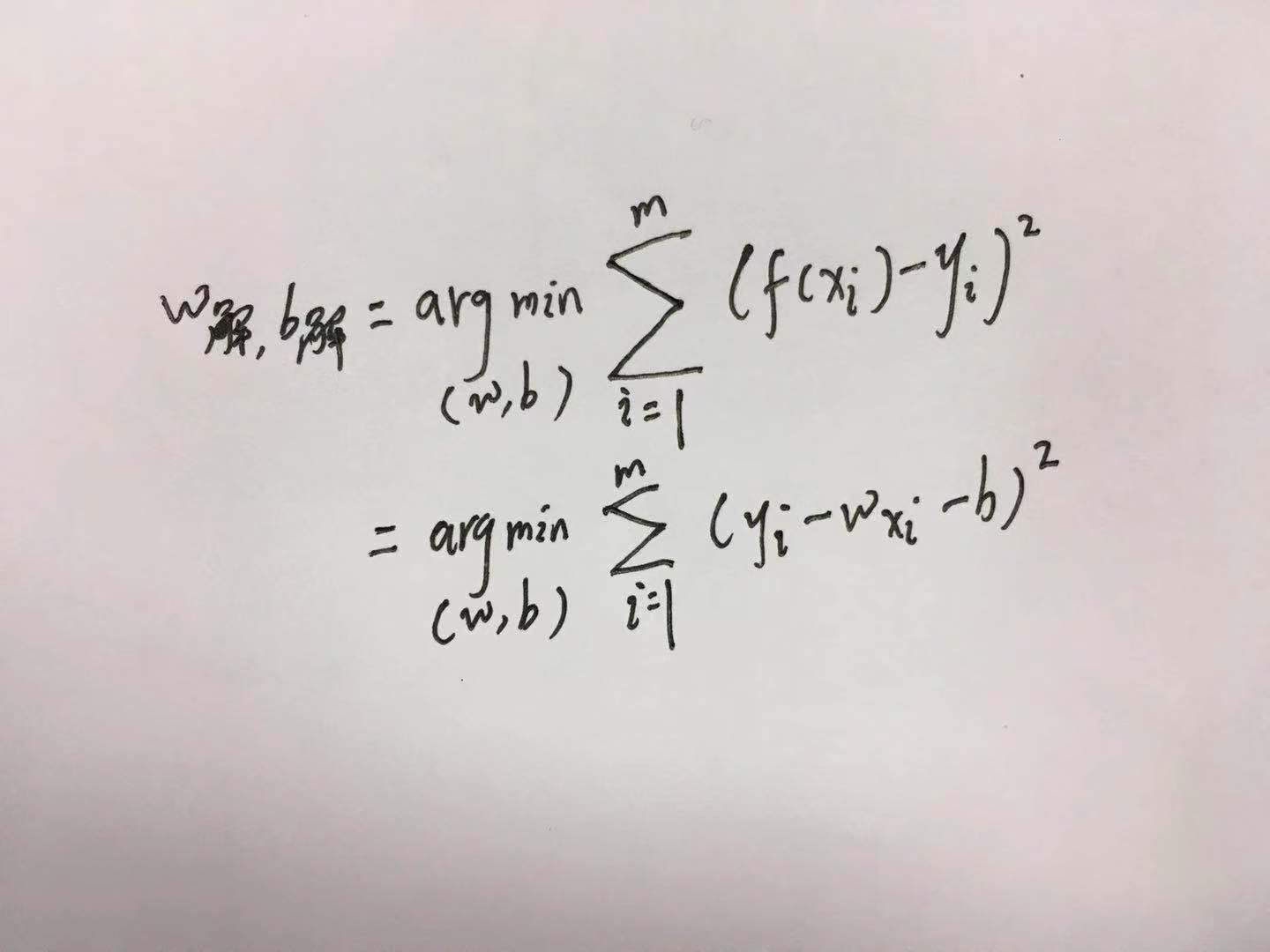这种基于均方误差最小化来进行线性模型求解的方法称为”最小二乘法”(least square method),线性回归中最小二乘法就是找到一条直线，使得所有样本到直线上的欧氏距离最小。
这个求解过程也被称为线性回归的最小二乘参数估计。
E ( w , b ) = ∑ i = 1 m ( y i − w x i − b ) 2 E_{(w,b)} = \sum_{i=1}^m(y_i - wx_i - b)^2

∂ L ( w , b ) ∂ w = − 2 ∑ i = 1 m ( x i y i − w x i 2 − b x i ) \frac{\partial L(w,b)}{\partial w} = -2 \sum_{i=1}^m(x_iy_i - wx_i^2 - bx_i)
我们知道形如 y = x 2 y=x^2 函数通常是凸函数，且极值点在导数为0时取得。
于是我们分别对 w w b b 求导得到: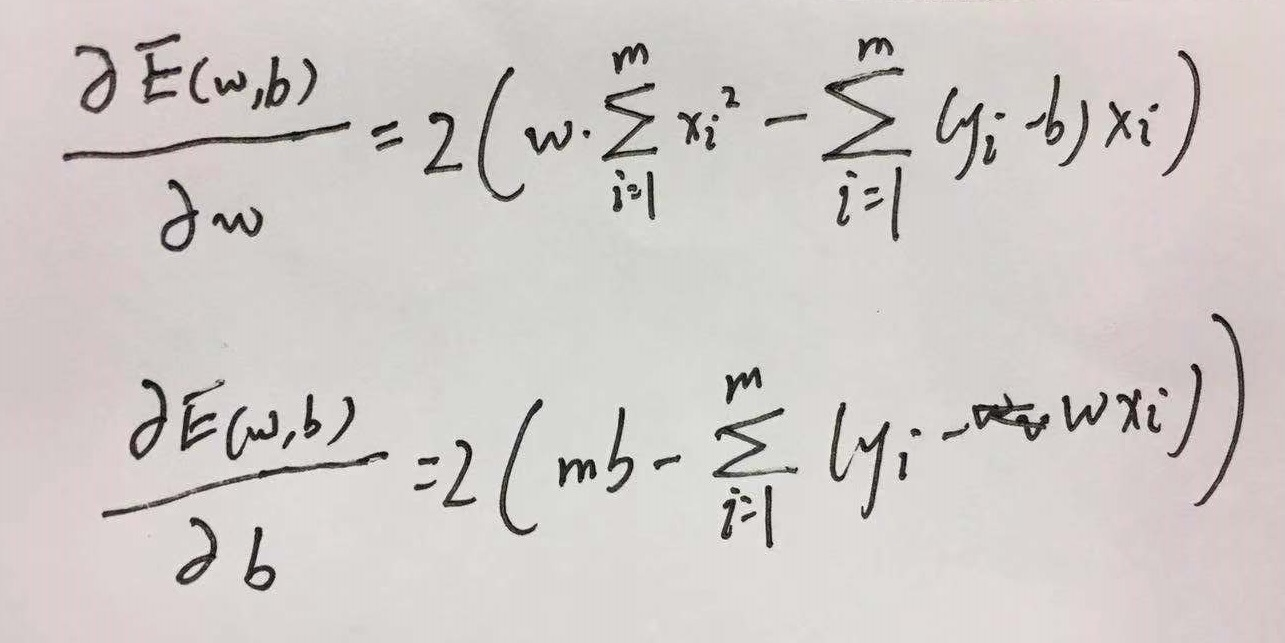令二式均为0就可以得到w与b最优解的闭式解：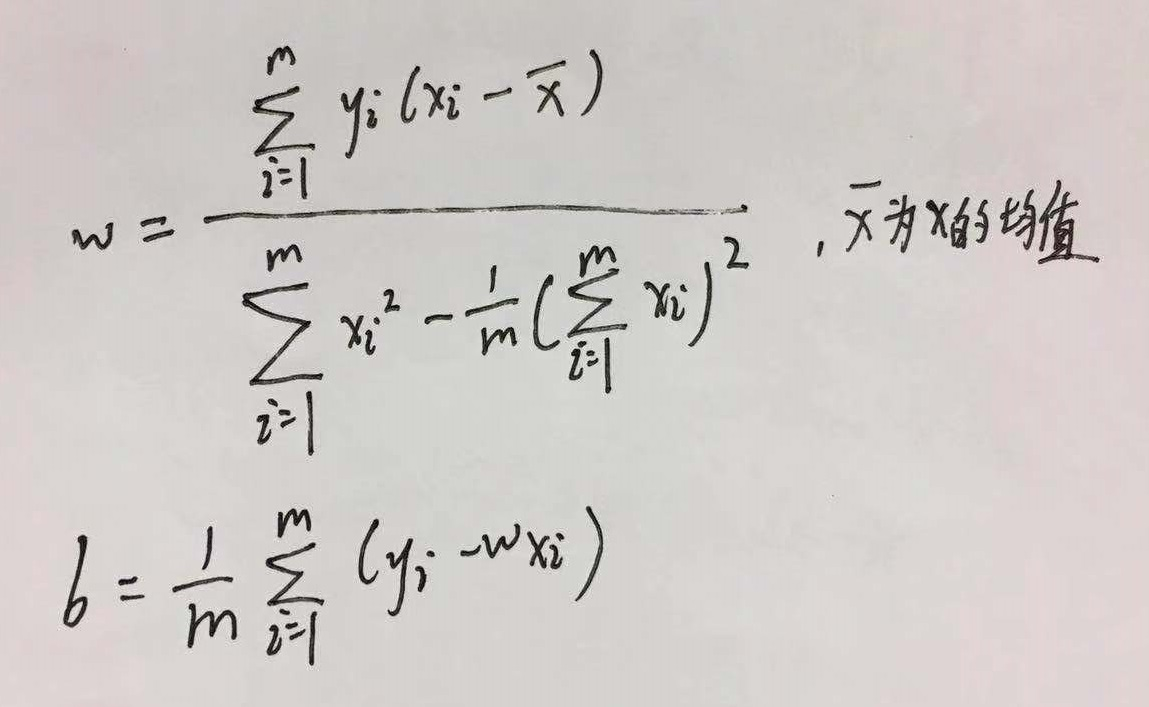这里可以提一下什么是闭式解，闭式解其实就是解析解，与数值解相对应，最笼统的解释解析解就是严格按照公式推导出来的解，而数值解是按照特定条件近似计算得到的基本满足的数值，逼近解析解，但是正确性没有解析解来的好。
那么当我们的输入是多个变量的时候该怎么办呢。
其实就是把 w w x x 向量化，即我们试图学得 f ( x i ) = w T x i + b f(\mathbf x_i)=\mathbf w^T\mathbf x_i+b ,使得 f ( x i ) ≈ y i f(\mathbf x_i)\approx y_i ，这就是多元线性回归
我们同样利用最小二乘法对 w w b b 进行估计。我们将 w w b b 结合得到 w ^ = ( w ; b ) \hat \mathbf w =(\mathbf w;b) ,同时把数据集D表示为一个 m X ( d + 1 ) mX(d+1) 大小的矩阵X 最后一个元素恒为1。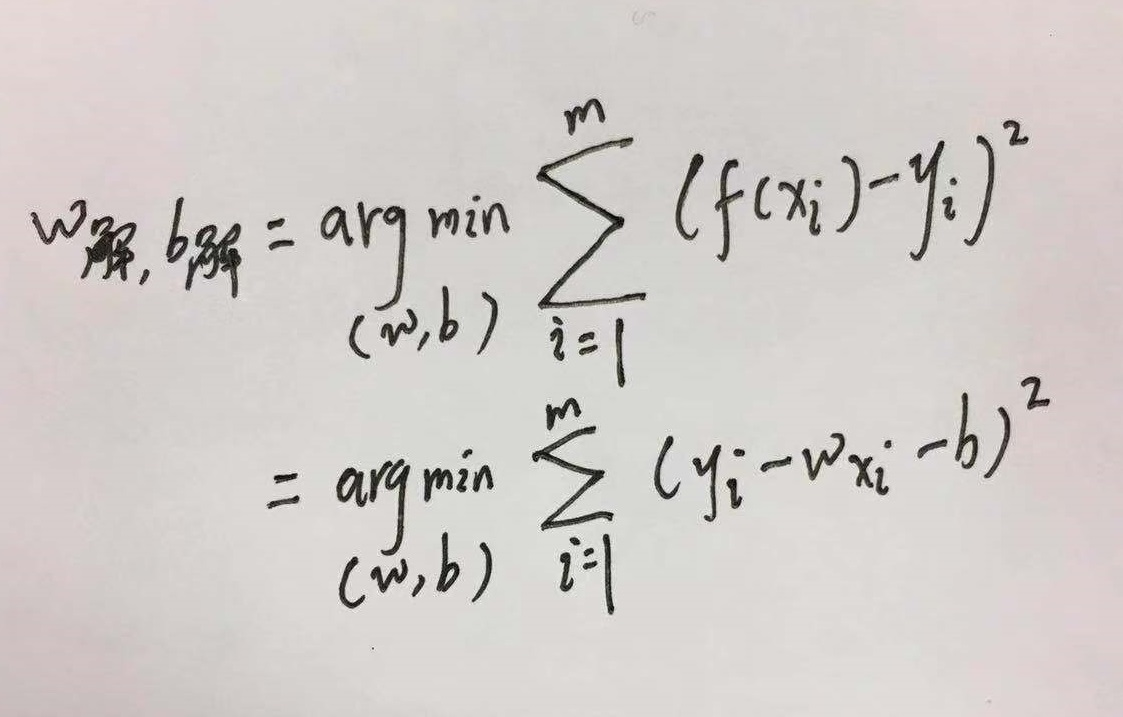于是上图可以化为：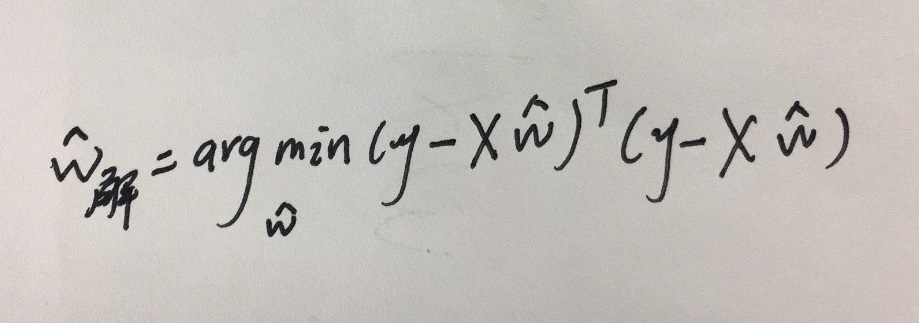E w ^ = ( y − X w ^ ) T ( y − X w ^ ) E_{\hat w}=(\mathbf y-\mathbf X\hat \mathbf w)^T(\mathbf y-\mathbf X\hat \mathbf w) ,对 w ^ \hat \mathbf w 求导，推导过程如下：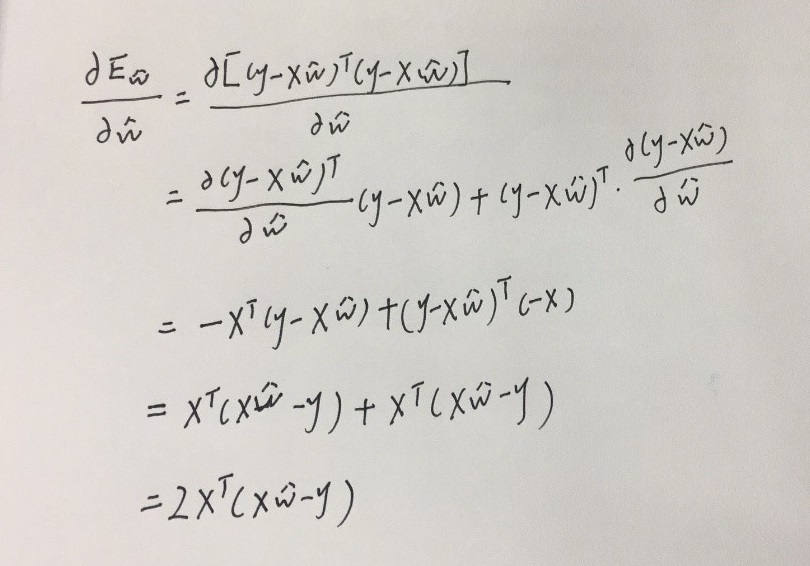只有当 X T X \mathbf X^Τ \mathbf X 为满秩矩阵或者正定矩阵时，令上式为0可以求得唯一解，但是现实中，我们碰到的往往都不满足满秩矩阵或者正定矩阵的情况，因此我们可以求得多个 w ^ \hat \mathbf w 使得均方误差最小，常见的方法是引入正则项化（下次再议）
当然线性模型会有很多种变形如：
ln ⁡ y = w T x i + b \ln y=\mathbf w^T\mathbf x_i+b

其实就是就是一种让输出标记在指数尺度上变化的形式，被称为对数线性回归。

# 2.对数几率回归

虽然名字叫做回归。但是千万别把他看成一个回归问题，对数几率回归本质上处理的分类问题。考虑最基本的二分类问题。那么我们的理想输出应该是1或0，即是或者不是。但是知道线性模型

f = w T x + b f=\mathbf w^T\mathbf x+b
产生的预测值是一个实数，我们只要想一个办法将预测值转化成为1或者0两个值，是不是就可以将回归问题转化为分类问题了呢？
怎么转化呢，最简单的方式肯定是分段函数直接截取最后预测值进行分段，如下所示
y = { 1 , if  f  &gt; 0 0.5 , if  f  = 0 0 , if  f  &lt; 0 y = \begin{cases} 1, &amp; \text{if $f$ &gt; 0} \\ 0.5, &amp; \text{if $f$ = 0} \\ 0, &amp; \text{if $f$ &lt; 0} \\ \end{cases}
f f 大于0则 y = 1 y=1 ，即为正例，若 f f 小于0则 y = 0 y=0 ，即为反例，若 f = 0 f=0 y = 0.5 y=0.5 ，即可以任意判别此例。
这里你们肯定不经想问为什么是以预测值 f f 大于小于等于0作为分界线的？让我们回到最初的线性方程，如下图所示,根据房间大小预测房价。我们可以看到当 f = 0 f=0 的时候，其实是预测点刚好落在这条线上，在分类问题中这条线我们称之为分界线。我们任意取一个输入 x = a x=a ，画一条竖线，我们可以看到分界线把 x = a x=a 分成了三部分，上方，下方，和相交的那一点。在上方的点对于这条预测线来说，价格都是偏高的，因此可以归类为价格高这个属性即 y = 1 y=1 而下方都是偏低的，归类为 y = 0 y=0 ，而在这条预测线上的点，我们无法定义他的价格属于高还是低，因此可以作为任意判别。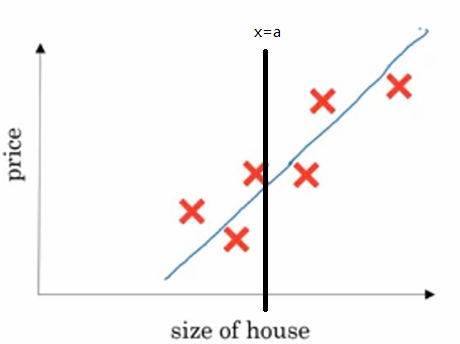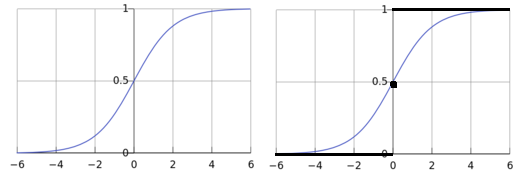再回到那个分段函数，我们把这种非常理想的函数形式称为“单位阶跃函数”(Heaviside函数)如上图黑线黑点所示。但是我们不难发现这种函数是不连续的，不可微，不可微带来的问题就是我们不能求解最佳的 w w b b 。那么我们就想呀，我们要找到一种形似单位阶跃函数却又单调可微的函数，那多简单，让它弯曲起来呀，于是我们就找到了上右图蓝色的函数形式，这个就是对数几率函数，它的解析式为：
y = 1 1 + e − f y = {1 \over 1 + e^{-f}}
从函数形式上不难看出，它将f值转化为一个接近1或者0的y值，并且让f=0附近的时候变化很剧烈。这部分不就是分界线附近的时候嘛，根据这个特点，不难看出来这个函数可以让我们更好的完成分类问题。
我们将之前 f f 的完整式带入得到:

y = 1 1 + e − f = 1 1 + e − ( w T x + b ) y = {1 \over 1 + e^{-f}} = {1 \over 1 + e^{-(w^Tx+b)}}
做一个对数形式的变换可以得到:
l n y 1 − y = w T x + b ln{y \over 1 - y} = w^Tx + b

回到我们最初的定义，我们将 y y 定义为测试样本 x x 是正例的可能性（越大就越可能是正例，1的时候一定是），反之 1 − y 1-y 就是测试样本 x x 为反例的可能性。那么两者的比值就更加显化了测试样本 x x 为正例的相对可能性，因为当比值大于1的时候就是测试样本 x x 为正例的可能性要大于为反例的可能性，我们将这个比值称为几率:
y 1 − y y \over 1 - y

对几率取对数就得到了对数几率，由于对数与几率在对应区间内单调性相同，因此对数几率仍然反应了测试样本x为正例的相对可能性。
l n y 1 − y ln{y \over 1 - y}
因此我们只要确定最优的 w w b b 就可以找到那个最优的分类模型了。求最优解，就是求一个极值，我们在线性回归当中提到，一般的线性模型并不一定只有一个最优解，而严格的凸函数才有唯一的最优解
那么对数几率回归的目标函数
l n y 1 − y = w T x + b ln{y \over 1 - y} = w^Tx + b
是不是一个严格的凸函数呢？
证明一个函数是严格凸函数，我们可以从二阶导恒大于0入手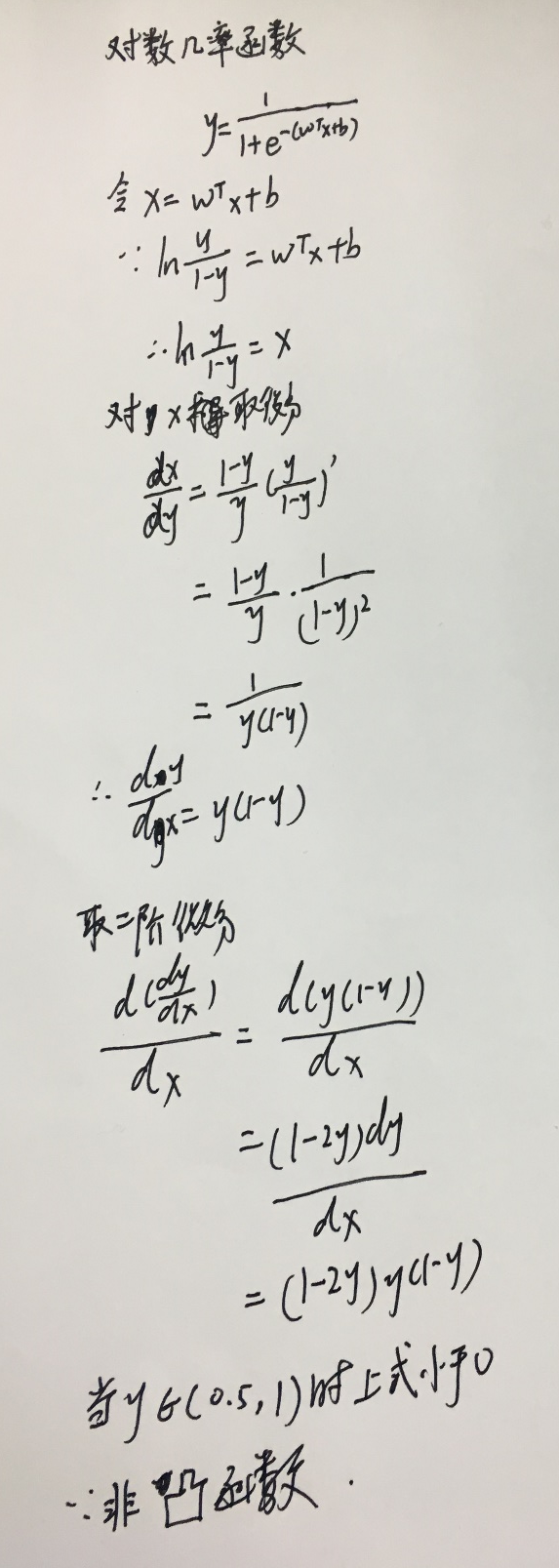那么我们该怎么求解 w w b b 呢？这个时候我们可以从极大似然法入手。首先我们将 y y 视为类后验概率估计 p = ( y = 1 ∣ x ) p=(y=1|x) ,于是得到：

{ y = p ( y = 1 ∣ x ) = e w T x + b 1 + e w T + b 1 − y = p ( y = 0 ∣ x ) = 1 1 + e w T + b \begin{cases} y &amp;= p(y=1 | x) = {e^{w^Tx + b} \over 1 + e^{w^T+b}} \\ 1 - y &amp;= p(y = 0 | x) = {1 \over 1 + e^{w^T+b}} \end{cases}
这时候我们可以用极大似然法来估计 w w b b 。对于给定的数据集 ( x i , y i ) i = 1 m {(x_i,y_i)}_{i=1}^m
那么对数几率回归模型最大化的对数似然为：

ℓ ( w , b ) = ∑ i = 1 m l n p ( y i ∣ x i ; w , b ) \ell_{(w, b)} = \sum_{i = 1}^mlnp(y_i | x_i; w,b)
为了便于讨论，令 Q = ( w , b ) , w ^ = ( w ; 1 ) Q=(w,b),\hat w =(w;1) ,则 w ^ T x + b \hat w^Tx+b 可简写为 Q T x ^ Q^T \hat x ,再令 p 1 ( w ^ ; Q ) = p ( y = 1 │ w ^ ; Q ) , p 0 ( w ^ ; Q ) = p ( y = 0 │ w ^ ; Q ) = 1 − p 1 ( w ^ ; Q ) p_1 {(\hat w ;Q)}=p(y=1│\hat w ;Q),p_0 (\hat w ;Q)=p(y=0│\hat w;Q)=1-p_1 (\hat w;Q) 上式的概率部分可以重写为：
p ( y i ∣ x i ; w , b ) = p 1 ( x i ^ ; Q ) y i p 0 ( x i ^ ; Q ) 1 − y i p(y_i | x_i; w,b)=p_1(\hat {x_i};Q)^{y_i}p_0(\hat {x_i}; Q)^{1 - y_i}

带入到对数似然式子中得到：
ℓ ( w , b ) = ∑ i = 1 m ( − y i Q T x ^ i +   l n ( 1 + e Q T x ^ i ) ) \ell_{(w, b)} = \sum_{i = 1}^m(-y_iQ^T\hat x_i+\ ln(1+\boldsymbol e^{Q^T\hat x_i}))
最大化最上面对数似然等价于最小化上式。但是这个时候有个前提，那就是上式是个严格凸函数才有唯一最小值。能不能证明呢？当然能啦，证明过程如下：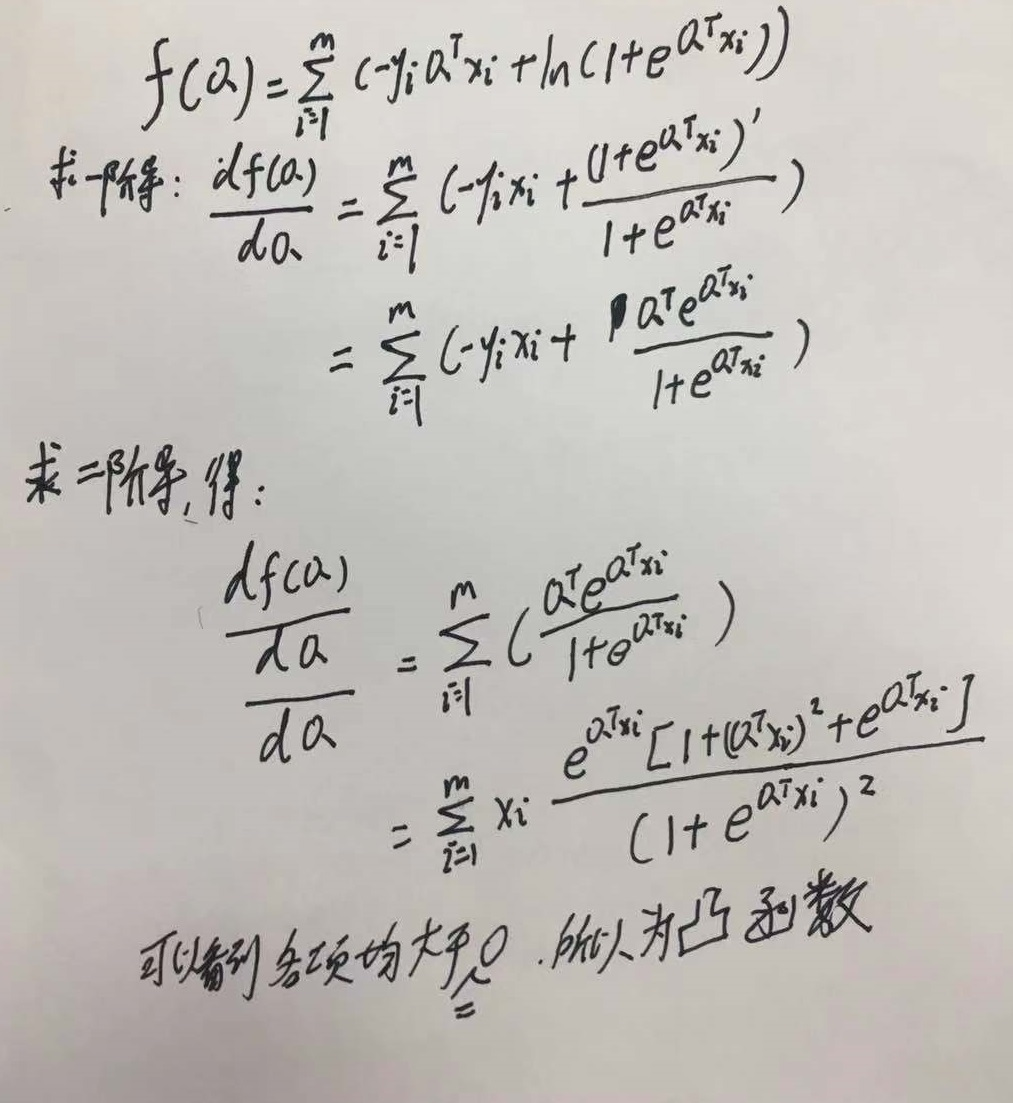根据凸优化理论，可以利用梯度下降法或者牛顿法等数值优化算法来求Q的最优解。

展开全文• 1.3、简单线性回归   前面提到过，算法说白了就是公式，简单线性回归属于一个算法，它所对应的公式。   ????=????????+????y=wx+b   这个公式中，y 是目标变量即未来要预测的值，x 是影响 y 的因素，w,b 是...线性回归 机器学习
• 如果只有一个自变量X，而且因变量Y和自变量X之间的数量变化关系呈近似线性关系，就可以建立一元线性回归方程，由自变量X的值来预测因变量Y的值，这就是一元线性回归预测。 数据： 拟合数据如下，我们要根据国内国内...python 机器学习
•线性回归
• 我总是告诉学生，采用自然对数来转换变量的三个原因。记录变量的原因将决定您是要记录独立变量还是因变量或两者。为了清楚起见，我正在谈论采用自然对数。首先，如其他海报所指出的那样，提高模型拟合度。例如，如果...
• python获取留存曲线的幂函数系数并计算生命周期LT，中介绍了获取幂函数系数的两种方式，下文对第一种方法【lnx、lny对应的线性拟合，最小二乘法求解系数】进行公式推导。python 线性回归
• 线性回归是一种研究影响关系的方法，在实际研究里非常常见。本文就来梳理下线性回归分析的分析流程，闲话少说，我们开始吧！线性回归回归分析实质上就是研究一个或多个自变量X对一个因变量Y(定量数据)的影响关系情况...
• 一元线性回归分析是一种非常简单也是非常基本的回归理论，能够用来刻画两个变量之间的以线性关系的变化趋势，进而预测未知点处的数据。本文以y = 2x + 1的数据进行拟合为例，给出了基于矩阵运算来估计系数的方法及...线性回归 matlab
• 线性回归方程3. 误差项分析4. 似然函数求解5. 线性回归求解 线性回归，是利用数理统计中回归分析，来确定两种或两种以上变量间相互依赖的定量关系的一种统计分析方法，运用十分广泛。 优点：结果易于理解，计算不...线性回归 机器学习
• 常用的三个线性模型的原理及python实现——线性回归（Linear Regression）、对数几率回归（Logostic Regression）、线性判别分析（Linear Discriminant）。 这可能会是对线性模型介绍最全面的博客一、线性模型 ...python 线性回归 lda算法原理
• 线性回归线性回归的延伸，线性就是每个变量的指数都是 1，而非线性就是至少有一个变量的指数不是 1。生活中，很多现象之间的关系往往不是线性关系。选择合适的曲线类型不是一件轻而易举的工作，主要依靠专业知识...非线性回归
• } } /----------- 线性方程 b,k,y ------------------------/ public decimal get_zxfc_k(List<> ls) { decimal k = 0; var ax = ls.Average(s => s.x); var ay = ls.Average(s => s.y); decimal fm = 0; decimal ...java 机器学习 python unity
• 线性回归算法 线性回归算法类型: 线性回归算法属于有监督学习的回归算法，可以处理标签为连续数据类型的数据。 线性回归算法原理: 通过寻找特征和标签之间的关系，生成一个线性方程，通过线性方程预测未知标签。 ...python sklearn 线性回归
• 并不是大的量和小的量放在一起大的就要对数，那我必然要问你，为什么不选择开N次方呢？或者把小的求阶乘(当然这种操作可能也不太“自然”)？事实上选择如何处理特征是要有一定的“具象”理由的，常见的两个原因有：...
• 线性回归，针对线性数据，通过最小二乘法让损失函数（cost function）最小，求得方程系数，得到方程；多元线性回归求解cost function：正规方程法和梯度下降；准线性回归的延伸：岭回归和Lasso回归；梯度下降法的...机器学习 线性回归
• 机器学习线性回归logistic回归 线性回归 线性回归是机器学习中比较基本的一个算法。其基本思想大致可以理解为给定一个数据集，通过线性回归得到一条曲线，尽可能地去拟合这个数据集。如下图所示： 那么，如何得到...线性回归 机器学习
• ## 线性回归--公式推导

万次阅读 多人点赞 2018-11-01 10:11:16
线性回归（Linear regression）是利用称为线性回归方程的最小二乘函数对一个或多个自变量和因变量之间关系进行建模的一种回归分析 —维基百科   线性回归--理论推导 &lt;1&gt; 数据: 本次推导使用的...机器学习 线性回归 大数据
• 文章目录通用形式简介重要概念指数族分布线性回归简介正则化基扩展和核函数对数几率回归简介多分类策略凸函数求解一些问题# 参考链接 通用形式 简介 ​ 广义线性模型（Generalized Linear Model），将线性模型...机器学习 逻辑回归
• Python实现线性回归实现目标实验数据结果分析源代码（更新中） 实现目标 1.实现一元（或多元）线性回归 a. 根据对客观现象的定性认识初步判断现象之间的相关性 b. 绘制散点图 c. 进行回归分析，拟合出回归模型 d. 对...python 线性回归
• 机器学习 线性回归 逻辑回归 非线性模型 R语言实现线性回归机器学习 线性回归
• 1 代价函数实现(cost function) function J = computeCost(X, y, theta)%COMPUTECOST Compute cost for linear regression% J = COMPUTECOST(X, y, theta) computes the cost of using theta as the% parameter for ...
• Python线性回归实战分析这篇文章主要介绍Python线性回归实战分析以及代码讲解，对此有兴趣的朋友学习下吧。一、线性回归的理论1)线性回归的基本概念...线性回归可以用来预测和分类，从回归方程可以看出自变量和因变...
• 生成SAS数据集（2）掌握用proc reg过程对数据进行一元线性回归分析和可转化一元线性的回归分析（3）会正确阅读一元回归分析的运行结果，并写出回归方程。 实验程序： 代码1： proc import out=test1 ...统一建模语言
• BG：在box-cox变换中，当λ = 0时即为对数变换。当所分析变量的标准差相对于均值而言比较大时，这种变换特别有用。对数据作对数变换常常起到降低数据波动性和减少不对称性的作用。。这一变换也能有效消除异方差性...
• 多元线性回归 回归分析： 通过研究自变量X和因变量Y的相关关系，尝试去解释Y的形成机制，进而达到通过X去预测Y的目的。 本次主要学习线性回归。（划分依据是因变量Y的类型） ps. 其他： 0-1回归，定序回归，计数回归...线性回归 多元回归...